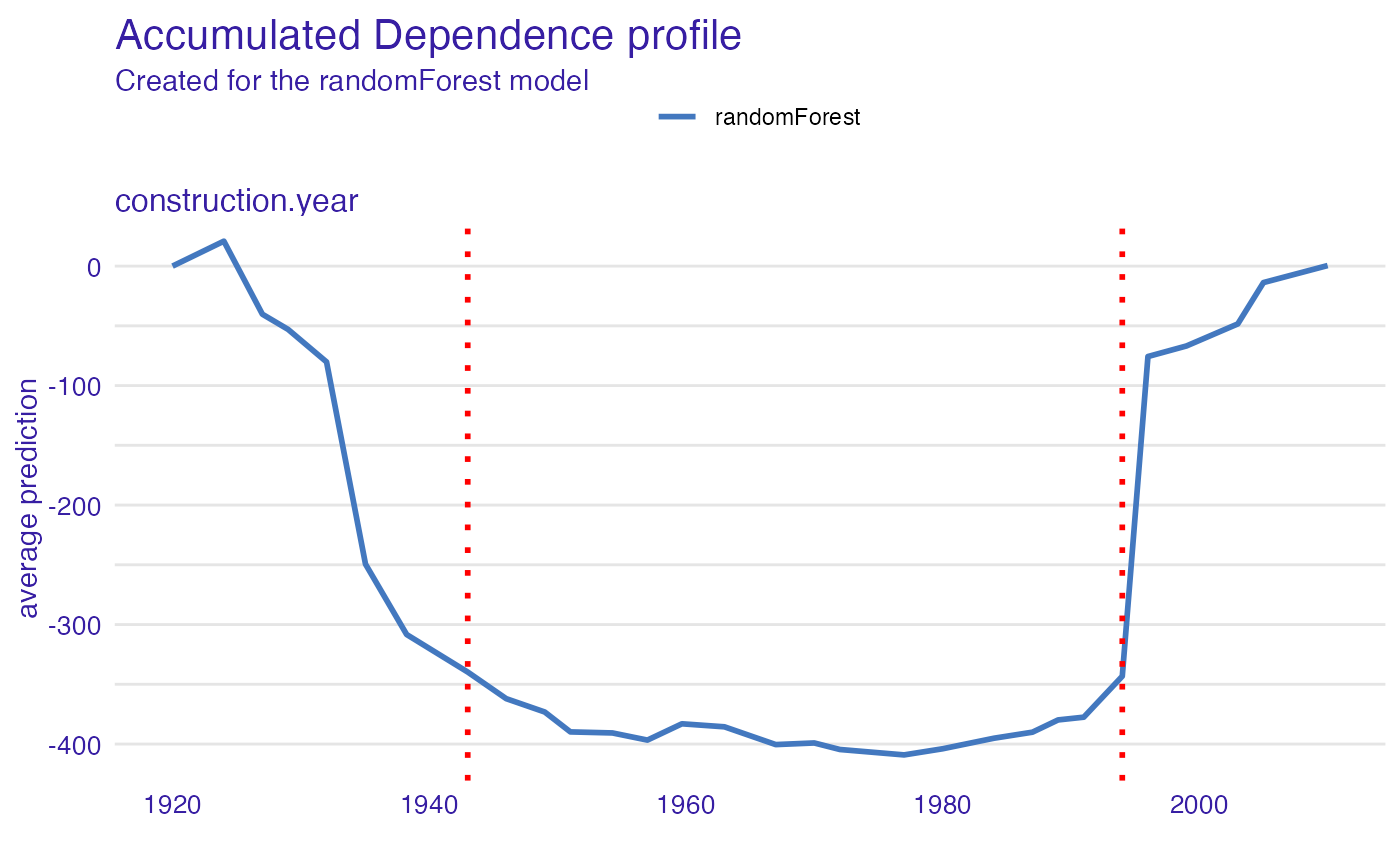The safe_extraction() function creates a SAFE-extractor object which may be used later for surrogate feature extraction.

safe_extraction(
explainer,
response_type = "ale",
grid_points = 50,
N = 200,
penalty = "MBIC",
nquantiles = 10,
no_segments = 2,
method = "complete",
B = 500,
collapse = "_",
interactions = FALSE,
inter_param = 0.25,
inter_threshold = 0.25,
verbose = TRUE
)

## Arguments

explainer DALEX explainer created with explain() function character, type of response to be calculated, one of: "pdp", "ale". If features are uncorrelated, one can use "pdp" type - otherwise "ale" is strongly recommended. number of points on x-axis used for creating the PD/ALE plot, default 50 number of observations from the dataset used for creating the PD/ALE plot, default 200 penalty for introducing another changepoint, one of "AIC", "BIC", "SIC", "MBIC", "Hannan-Quinn" or numeric non-negative value the number of quantiles used in integral approximation numeric, a number of segments variable is to be divided into in case of founding no breakpoints the agglomeration method to be used in hierarchical clustering, one of: "ward.D", "ward.D2", "single", "complete", "average", "mcquitty", "median", "centroid" number of reference datasets used to calculate gap statistics a character string to separate original levels while combining them to the new one logical, if interactions between variables are to be taken into account numeric, a positive value indicating which of single observation non-additive effects are to be regarded as significant, the higher value the higher non-additive effect has to be to be taken into account numeric, a value from [0,1] interval indicating which interactions should be returned as significant. It corresponds to the percentage of observations for which interaction measure is greater than inter_param - if this percentage is less than inter_threshold then interaction effect is ignored. logical, if progress bar is to be printed

## Value

safe_extractor object containing information about variables transformation

safely_transform_categorical, safely_transform_continuous, safely_detect_interactions, safely_transform_data

## Examples


library(DALEX)
#> Welcome to DALEX (version: 2.2.1).
#> Find examples and detailed introduction at: http://ema.drwhy.ai/
library(randomForest)
#> randomForest 4.6-14
#> Type rfNews() to see new features/changes/bug fixes.
library(rSAFE)

data <- apartments[1:500,]
set.seed(111)
model_rf <- randomForest(m2.price ~ construction.year + surface + floor +
no.rooms + district, data = data)
explainer_rf <- explain(model_rf, data = data[,2:6], y = data[,1], verbose = FALSE)
safe_extractor <- safe_extraction(explainer_rf, grid_points = 30, N = 100, verbose = FALSE)
print(safe_extractor)
#> Variable 'construction.year' - selected intervals:
#> 	(-Inf, 1943]
#>  	(1943, 1994]
#>  	(1994, Inf)
#> Variable 'surface' - selected intervals:
#> 	(-Inf, 47]
#>  	(47, 101.7241]
#>  	(101.7241, Inf)
#> Variable 'floor' - selected intervals:
#> 	(-Inf, 5]
#>  	(5, Inf)
#> Variable 'no.rooms' - selected intervals:
#> 	(-Inf, 2]
#>  	(2, Inf)
#> Variable 'district' - created levels:
#> 	Bemowo, Bielany, Ursus, Ursynow, Praga, Wola ->  Bemowo_Bielany_Praga_Ursus_Ursynow_Wola
#> 	Zoliborz, Mokotow, Ochota ->  Mokotow_Ochota_Zoliborz
#> 	Srodmiescie ->  Srodmiescie
plot(safe_extractor, variable = "construction.year")